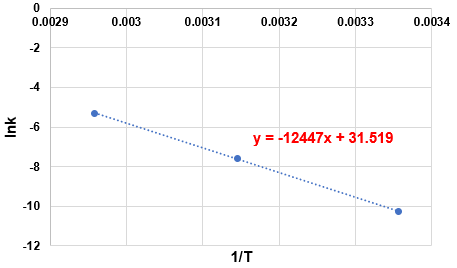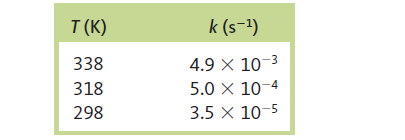# Problem: The rate constant for the gas-phase decomposition of N2O5,N2O5 → 2NO2 + 1/2 O2has the following temperature dependence:Make the appropriate graph using these data, and determine the activation energy for this reaction.

###### FREE Expert Solution

The data given is plotted by lnk (y) vs. 1/T (x).91% (338 ratings)###### Problem Details

The rate constant for the gas-phase decomposition of N2O5,

N2O5 → 2NO2 + 1/2 O2

has the following temperature dependence:Make the appropriate graph using these data, and determine the activation energy for this reaction.